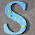## Thursday, June 12, 2014

### Basic Electrical objective type questions: Part-13

Electrical Objective Questions with Answers:

 In a uniform electric field, field lines and equipotentials [Gate 1997 ]

a) Are parallel to one another
b) Intersect at 45 deg
c) Intersect at 30 deg
d) Are orthogonal

 For a pulse transformer, the material used for its core and the possible turn ratio from primay to secondary are respectively
(a) ferrite; 20:1
(b) laminated iron; 1:1
(c) ferrite; 1:1
(d) powdered iron; 1:1

 The capacitance of a reverse biased junction of a thyristor is 20 picofarad. The charging current of this thyristor is 4mA. The limiting value

of dv/dt is .....V/uS

 When the induction motor runs at synchronous speed the voltage induced in the rotor of it is

(a) Very near input voltage to stator
(b) Slip time the input voltage
(c) Zero
(d) None of the above

 Chopper control for the DC motor provides variation in

(a) Input voltage
(b) Frequency
(c) None of the above

 While comparing SCR with BJT, which of the following is true

(a) SCR requires turn off circuit while BJT does not
(b) The voltage drop of SCR is less than BJT
(c) SCR requires a continuous gate current
(d) BJT draws continuously base current
Which of the above statements are true?
(1) a only
(2) d only
(3) a, d
(4) b, d

Common Data Questions: 7 & 8
In the 3-phase inverter circuit shown, the load is balanced and the gating scheme is 180°-conduction mode.All the switching devices are idealThe rms value of load phase voltage is [GATE2012]

a. 106.1V
b. 141.4V
c. 212.2V
d. 282.8V

 If the dc bus voltage Vd=300V, the power consumed by 3-phase load is
[GATE2012]
a. 1.5kW
b. 2.0kW
c. 2.5kW
d. 3.0kW

 The typical ratio of latching current to holding current in a 20A thyristor is [GATE 2012]
a. 5.0
b. 2.0
c. 1.0
d. 0.5

 A half-controlled single-phase bridge rectifier is supplying an R-L load. It is operated at a firing angle  α and the load current is continuous. The fraction of cycle that the freewheeling diode conducts is [GATE 2012]
a. 1/2
b. (1-α/π)
c. α/2π
d. α/π


For power factor correction of power systems which one is used:

(a) capacitor
(b) inductor
(c) resistors

 Which type of motor used in home ceiling fan:

(a) capacitor motor
(b) shaded pole motor
(c) universal motor
(SPLIT PHASE CAPACITOR MOTOR)

 Op-amp (operational amplifier) input resistance:

(a) Zero
(b) Infinity
(c) Relative resistance
(d) Same output resistance

 For an inverter op-amp, what will be the equivalent output resistance?

(a) Zero
(b) Infinity
(c) Relative forward resistance
(d) Same as the input resistance
9:31 AM

#### 6 comments:

1.Q: which application DC motor preffer on AC motor?
1)variable speed operation
2)high speed
3)low speed
4)fixed speeed

plz give ans

1.FIXED SPEED

2.High speed because of high stating torque in dc motor

3.FIXED SPEED *BIM 10.01.2016

4.All are possible
But in ac motor syn motor type fixed speed
In Im variable speed drives used
In more application we use low speed drives
Ans is : 3

5.Variable speed operation because d.c motora speed control is good as compared a.c motors.